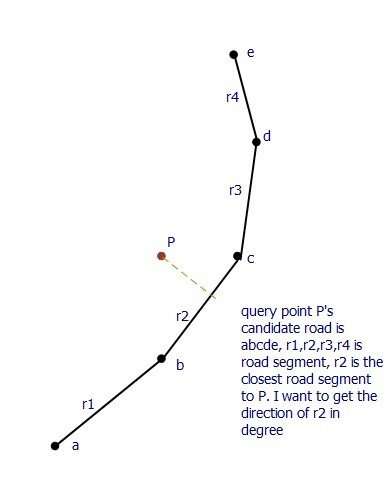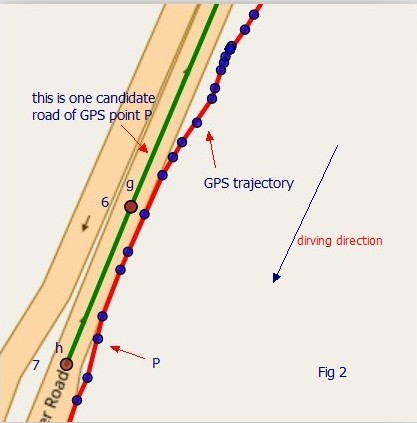# How to get the direction of a road segment in degree?

Hi, Peter and developers,

I am using Graphhopper to do map matching. I get the query point’s candidates by calling

``````  locationIndex.findNClosest(gpxEntry.getLat(), gpxEntry.getLon(), edgeFilter, measurementErrorSigma);
``````

It will return a list of QueryResult, each QueryResult has

``````EdgeIteratorState closestEdge,
``````

many short road segment is not a straight line. Now I want to get the
direction of the road segment which is closest to the query point in degree.
Maybe a figure is necessaried to better discribe my problem. Thanks a lot.1 Like

You can call `fetchWayGeometry` for the edge you need. See also the documentation about the low level api

Thank you for your time, Peter. Yes, I do it like this:

``````PointList pl = qr.getClosestEdge().fetchWayGeometry(3);
``````

Here pl is a set of point a、b、c、d、e in above figure, am i right? But how to get the closest road segment r2’s start node b and end node c still confused me.

Can anybody help me?

I have a very slow solution which had been a bottlenech of my map matching algorhtm’s efficientcy.

Getting the closest edge is supposed to return the bc edge in the example.
And then fetching edge’s geometry would return its base and adjacent nodes.
What are you getting in your results?

Yes @devemux86 is right for the case that a junction is on all a,b,c,d,e.

Or is a junction just on a and e? If this is the case then you can get the road part and its angle with qr.getWayIndex() and the fetchWayGeometry method

Thank you for your time, Peter and @devemux86. You are right for this case.
Now I figure out what is wayIndex. And I want to present a real example from my experiment。Fig 1 is a full view of a candidate road, which has 8 points. I get it by:

``````PointList pl = qr.getClosestEdge().fetchWayGeometry(3);
``````

we know pl is a point list, so the numbe in Fig. 1 means the index of each point in pl and I give each point a name a - h to better describe it.

Fig 2 has two line, the red one is GPS trajectory, and green one pl is amplified candidate road in Fig. 1. Actually, it’s GPS point P’s candidate road.

We can see the driving direction of the car is different from pl’s point order, but sometimes they have the same the direction. This means I cannot descide the road direction based on the order of pl.

Here qr’s wayIndex is 6.

``````int wayIndex = qr.getWayIndex();
double lat = pl.pl.getLat(wayIndex);
double lon = pl.getLon(wayIndex);
``````

According the (lat,lon), I understand the wayIndex means point g’s index in pl. So the closest edge is gh. But you can see the road direction is h -> g, not g -> h. So in my code, I cannot decide the road direction only by the order of pl’s point.

Currently， I decide the road direction by compare the Dijkstra distance between g -> h and h -> g in the road network. If g -> h has smaller distance, then the road direction is g -> h, then I use g and h to get the angle of road. The problem is Dijkstra algorithm is very slow. And if the road is bi-directional, this method will failed.

So do you have any better idea?

1 Like

If you do this with the map matching module the edges should be already in the correct direction. I also think this algo is a better fit compared to manually doing this?